### Home > CCAA > Chapter 8 Unit 7 > Lesson CC2: 8.3.3 > Problem8-90

8-90.

Find the value of each variable in the following figures. Do not use a protractor. Use the properties of angles to help you.

1.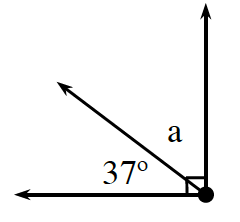$37°+a=90°$. Solve for $a$.

$53°$

1.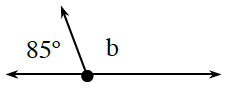These angles are supplementary.
$180°-85°=b$

1.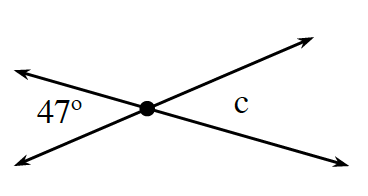$47°$ and $c$ are vertical angles. What does that tell you about the measure of angle $c$?

1.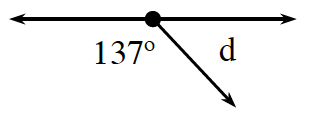Set up an equation relating $137°$ and $d$. $137°+d=180°$.

Subtract $137°$ from both sides.
$137°+d=180°\\ -137° \quad -137°\\ \qquad \quad d = 43°$

$43°$

1.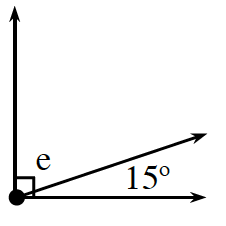$e$ and $15°$ are complementary angles, meaning their sum is $90°$.

1.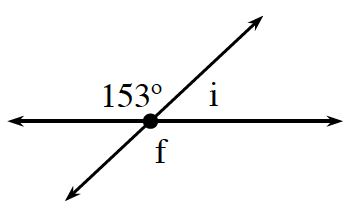$f$ and $153°$ are vertical angles, and $i$ and $153°$ are supplementary.

$f=153°$, $i=27°$

1.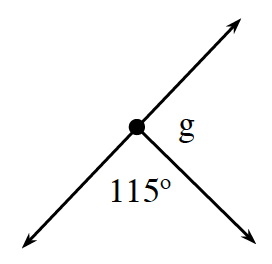$115°+g=180°$. Refer to part (d) for the steps to solving this problem.

1.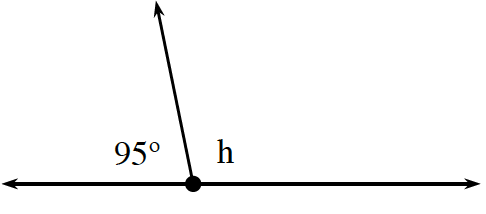$95°+h=180°$. Now solve for the value of $h$.

$85°$